# Recommendation System for Streaming Platforms Tutorial

In this Python tutorial, explore movie data of popular streaming platforms and build a recommendation system.
Aug 2020  · 16 min read

Due to the new culture of Binge-watching TV Shows and Movies, users are consuming content at a fast pace with available services like Netflix, Prime Video, Hulu, and Disney+. Some of these new platforms, such as Hulu and YouTube TV, also offer live streaming of events like Sports, live concerts/tours, and news channels. Live streaming is still not adopted by some of the streaming platforms, such as Netflix.

Streaming platforms provide more flexibility to users to watch their favorite TV shows and movies, at any time, on any device. These services can attract more young and modern consumers because of its wide variety of TV and movie content. It allows them to watch any missed program as their availability. In this tutorial, you will analyze movie data of streaming platforms Netflix, Prime Video, Hulu, and Disney+ and try to understand their viewers.## Understanding the Dataset

This data consisted of only movies available on streaming platforms such as Netflix, Prime Video, Hulu, and Disney+. You can download it from Kaggle here.

Let's describe data attributes in detail:

• ID: It is a unique ID for each record.
• Title: It is the name of the movie
• Year: Release year of the movie.
• Age: it is the target age group
• IMDb: IMDB rating of movies.
• Rotten Tomatoes: Rotten Tomatoes %
• Netflix: whether the movie is found on Netflix
• Hulu: whether the movie is found on Hulu
• Prime Video: whether the movie is found on Prime Video
• Disney+: whether the movie is found on Disney+
• Type: Movie or TV show
• Directors: Name of the director
• Genres: Type of genre
• Country: Country of origin
• Language: Language of origin
• Runtime: Duration of the movie

Let's import the necessary modules and load the dataset:

`````` # Import required libraries
import numpy as np
import pandas as pd
import matplotlib.pyplot as plt
import seaborn as sns
from sklearn.feature_extraction.text import TfidfVectorizer
from nltk.tokenize import RegexpTokenizer
import numpy as np
from sklearn import preprocessing
from scipy.sparse import hstack
import pandas_profiling``````
``````# Load dataset
df=df.iloc[:,1:] # removing in unnamed index column``````
``df.head()``
Unnamed: 0 ID Title Year Age IMDb Rotten Tomatoes Netflix Hulu Prime Video Disney+ Type Directors Genres Country Language Runtime
0 0 1 Inception 2010 13+ 8.8 87% 1 0 0 0 0 Christopher Nolan Action,Adventure,Sci-Fi,Thriller United States,United Kingdom English,Japanese,French 148.0
1 1 2 The Matrix 1999 18+ 8.7 87% 1 0 0 0 0 Lana Wachowski,Lilly Wachowski Action,Sci-Fi United States English 136.0
2 2 3 Avengers: Infinity War 2018 13+ 8.5 84% 1 0 0 0 0 Anthony Russo,Joe Russo Action,Adventure,Sci-Fi United States English 149.0
3 3 4 Back to the Future 1985 7+ 8.5 96% 1 0 0 0 0 Robert Zemeckis Adventure,Comedy,Sci-Fi United States English 116.0
4 4 5 The Good, the Bad and the Ugly 1966 18+ 8.8 97% 1 0 1 0 0 Sergio Leone Western Italy,Spain,West Germany Italian 161.0
``````# Show initial information about the dataset
df.info()
``````
``````<class 'pandas.core.frame.DataFrame'>
RangeIndex: 16744 entries, 0 to 16743
Data columns (total 16 columns):
#   Column           Non-Null Count  Dtype
---  ------           --------------  -----
0   ID               16744 non-null  object
1   Title            16744 non-null  object
2   Year             16744 non-null  int64
3   Age              7354 non-null   object
4   IMDb             16173 non-null  float64
5   Rotten Tomatoes  5158 non-null   object
6   Netflix          16744 non-null  int64
7   Hulu             16744 non-null  int64
8   Prime Video      16744 non-null  int64
9   Disney+          16744 non-null  int64
10  Type             16744 non-null  int64
11  Directors        16018 non-null  object
12  Genres           16469 non-null  object
13  Country          16309 non-null  object
14  Language         16145 non-null  object
15  Runtime          16152 non-null  float64
dtypes: float64(2), int64(6), object(8)
memory usage: 2.0+ MB
``````
``````df.Type.unique()
``````
``````array()
``````

## Working with Missing Values

In this section, You will work with missing values using the `isnull()` function. Let's see an example below:

`````` #Finding Missing values in all columns
miss = pd.DataFrame(df.isnull().sum())
miss = miss.rename(columns={0:"miss_count"})
miss["miss_%"] = (miss.miss_count/len(df.ID))*100
miss``````
misscount miss%
ID 0 0.000000
Title 0 0.000000
Year 0 0.000000
Age 9390 56.079790
IMDb 571 3.410177
Rotten Tomatoes 11586 69.194935
Netflix 0 0.000000
Hulu 0 0.000000
Prime Video 0 0.000000
Disney+ 0 0.000000
Type 0 0.000000
Directors 726 4.335882
Genres 275 1.642379
Country 435 2.597946
Language 599 3.577401
Runtime 592 3.535595

You can see that the variables Age and Rotten tomatoes have more than 50 % missing values, which is alarming.

Now, we will handle the missing values in the following steps:

• Drop columns which has more than 50% missing values
• Drop NA from IMDb, Directors, Genres, Country, Language, and Runtime column
• Reset index
• Convert year column into object
``````# Dropping values with missing % more than 50%
df.drop(['Rotten Tomatoes', 'Age'], axis = 1, inplace=True)
# Dropping Na's from the following columns
df.dropna(subset=['IMDb','Directors', 'Genres', 'Country', 'Language', 'Runtime'],inplace=True)
df.reset_index(inplace=True,drop=True)
# Converting into object type
df.Year = df.Year.astype("object")``````
``df.info()``
``````<class 'pandas.core.frame.DataFrame'>
RangeIndex: 15233 entries, 0 to 15232
Data columns (total 14 columns):
#   Column       Non-Null Count  Dtype
---  ------       --------------  -----
0   ID           15233 non-null  object
1   Title        15233 non-null  object
2   Year         15233 non-null  object
3   IMDb         15233 non-null  float64
4   Netflix      15233 non-null  int64
5   Hulu         15233 non-null  int64
6   Prime Video  15233 non-null  int64
7   Disney+      15233 non-null  int64
8   Type         15233 non-null  int64
9   Directors    15233 non-null  object
10  Genres       15233 non-null  object
11  Country      15233 non-null  object
12  Language     15233 non-null  object
13  Runtime      15233 non-null  float64
dtypes: float64(2), int64(5), object(7)
memory usage: 1.6+ MB
``````

## Distribution Plots

You can check the distribution of the Year column using the `distplot()` function of seaborn. Let's plot the Movie Year distribution plot.

``````#checking Distribution of years
plt.figure(figsize=(20,5))
sns.distplot(df['Year'])
plt.show()
``````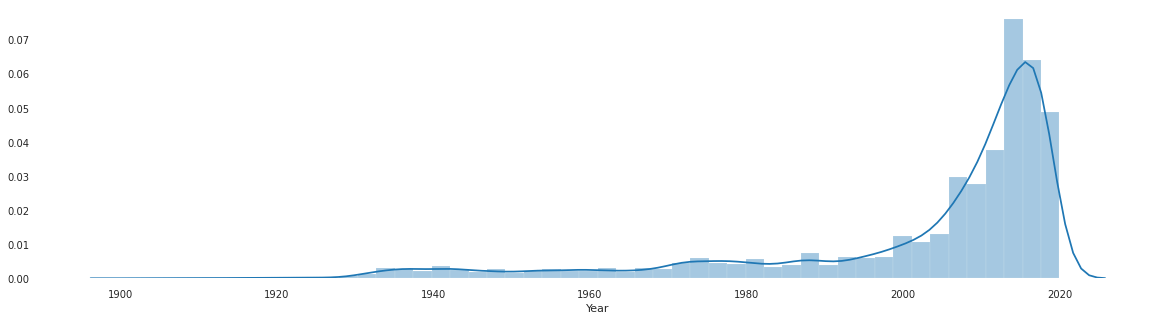The chart is showing the distribution of movies origin year. You can interpret that most of the movies were made between the year 2000 to 2020. Let's plot the IMDB rating distribution plot.

``````# Distribution of IMDb Rating
plt.figure(figsize=(20,5))
sns.distplot(df['IMDb'])
plt.show()
``````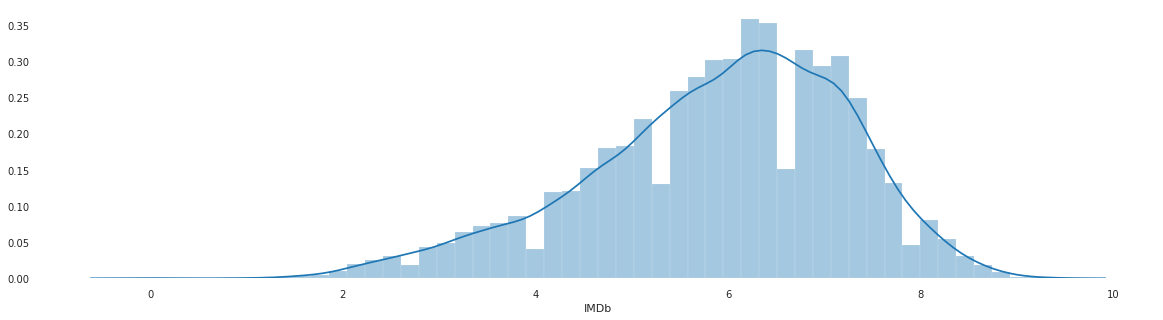The above distribution plot is slightly skewed. You can interpret that the mean IMDB of most movies is 6.5.

Let's plot the Movie Runtime distribution plot.

``````# Distribution of runtime
sns.distplot(df['Runtime'])
plt.show()
``````From the above chart, you can interpret that the movie's average runtime lies between 80 to 120 mins.

## Distribution of Movies on each Streaming Platform

In this section, you will see Streaming Platform wise movie distribution. First, you need to create an `m_cnt()` function that counts movies for a given streaming platform. After that, you can plot using Pie charts and understand the shares of streaming platforms.

``````# A function to calculate the movies in different Streaming platforms
def m_cnt(plat, count=False):
if count==False:
print('Platform {} Count: {}'. format(plat, df[plat].sum()))
else:
return df[plat].sum()
``````
``````# Let's see count of movies/shows of each streaming platform
m_cnt('Netflix')
m_cnt('Hulu')
m_cnt('Prime Video')
m_cnt('Disney+')
``````
``````Platform Netflix Count: 3152
Platform Hulu Count: 848
Platform Prime Video Count: 11289
Platform Disney+ Count: 542
``````
``````# Movies on each platform
lab = 'Prime Video','Netflix', 'Hulu', 'Disney'
s = [m_cnt('Prime Video', count=True),
m_cnt('Netflix', count=True),
m_cnt('Hulu', count=True),
m_cnt('Disney+', count=True)]

explode = (0.1, 0.1, 0.1, 0.1)

#plotting
fig1, ax1 = plt.subplots()
ax1.pie(s,
labels = lab,
autopct = '%1.1f%%',
explode = explode,
startangle = 100)

ax1.axis = ('equal')
plt.show()
``````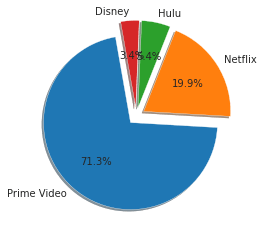From the above plot, you can say that Prime Videos is hosting the maximum number of titles with 71% share and Netflix hosting 20% of titles. Disney+ and Hulu are hosting the lowest titles, 5.4%, and 3.4%, respectively.

## Movie Distribution According to Genre

In this section, you will see genre-wise movie distribution. First, you need to prepare your data. You need to handle multiple genres given in a single cell of dataframe. For that, you can use `split()`, `apply()`, and `stack()` functions. `split()` function splits the multiple values with a comma and creates a list. `apply(pd.Series,1)` to create multiple columns for each genre and `stack()` function stack them into a single column.

After these three operations, a new Genres column will join with the existing dataframe, and you are ready to plot. You can show the top 10 genres with their movie count using `value_counts()` function and use `plot()` function of the pandas library.

`````` ##split the genres by ',' & then stack it one after the other for easy analysis.
g = df['Genres'].str.split(',').apply(pd.Series, 1).stack()
g.index = g.index.droplevel(-1)
# Assign name to column
g.name = 'Genres'
# delete column
del df['Genres']
# join new column with the existing dataframe
df_genres = df.join(g)``````
``````# Count of movies according to genre
plt.figure(figsize=(15,5))
sns.countplot(x='Genres', data=df_genres)
plt.xticks(rotation=90)
plt.show()``````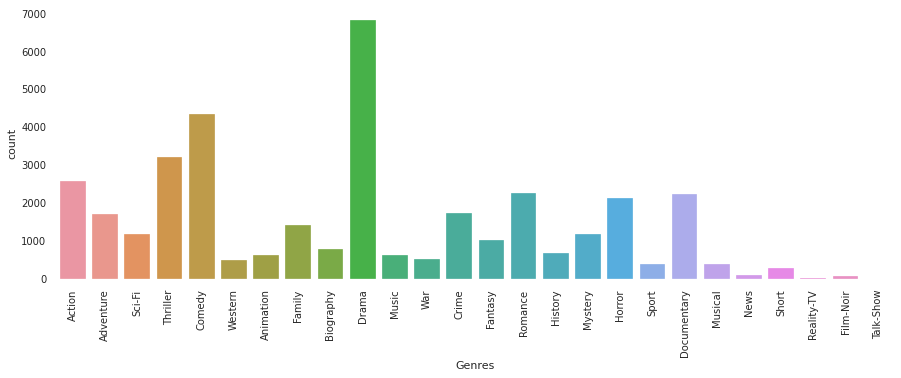From the above plot, you can say that most of the movies have a common genre as Drama and Comedy.

## Movie Distribution According to Country

In this section, you will see the country-wise movie distribution. First, you need to prepare your data. You need to handle multiple countries given in a single cell of dataframe. For that, you can use `split()`, `apply()`, and `stack()` functions. The `split()` function splits the multiple values with a comma and creates a list. `apply(pd.Series,1)` to create multiple columns for each country and `stack()` function stack them into a single column.

After these three operations, a new Country column will join with the existing dataframe and you are ready to plot. You can show the top 10 countries with their movie count using `value_counts()` function and use the pandas library's `plot()` function.

`````` # Split the Country by ',' & then stack it one after the other for easy analysis.
c = df['Country'].str.split(',').apply(pd.Series, 1).stack()
c.index = c.index.droplevel(-1)
# Assign name to column
c.name = 'Country'
# delete column
del df['Country']
# join new column with the existing dataframe
df_country = df.join(c)``````
``````# plotting top 10 country and movie count
df_country['Country'].value_counts()[:10].plot(kind='bar',figsize=(15,5))
plt.show()``````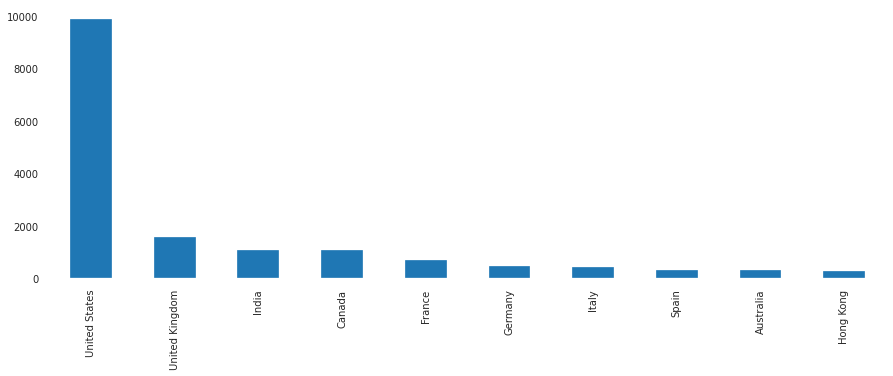The above graph shows that the majority of the movies were made in the United States.

## Movie Distribution According to Language

In this section, you will see language-wise movie distribution. First, you need to prepare your data. You need to handle multiple languages given in a single cell of the dataframe. For that, you can use `split()`, `apply()`, and `stack()` functions. `split()` function spits the multiple values with a comma and creates a list. `apply(pd.Series,1)` to create multiple columns for each language and `stack()` function stack them into a single column.

After these three operations, a new Language column will join with the existing dataframe, and you are ready to plot. You can show the top 10 languages with their movie count using `value_counts()` function and use the `plot()` function of the pandas library.

`````` # perform stacking operation on language column
l = df['Language'].str.split(',').apply(pd.Series,1).stack()
l.index = l.index.droplevel(-1)
# Assign name to column
l.name = 'Language'
# delete column
del df['Language']
# join new column with the existing dataframe
df_language = df.join(l)``````
``````# plotting top 10 Language and movie count
df_language['Language'].value_counts()[:10].plot(kind='bar',figsize=(15,3))
plt.show()``````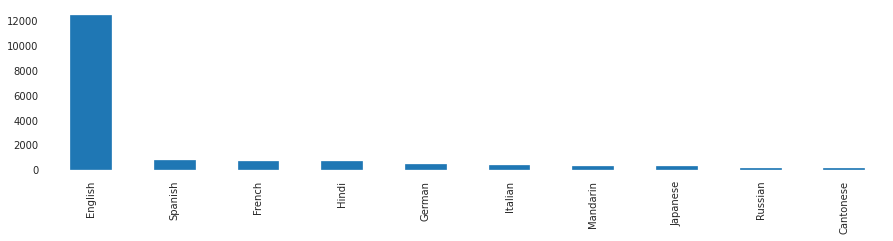From the above plot, you can conclude that the majority of movies were in the English language.

## IMDB rating Distribution According on each Platform

In this section, you will plot platform-wise IMDB rating distribution. For getting these results, you need to apply the `melt()` function and plot FacetGrid plot. `melt()` function converts a wide dataframe to a long dataframe. Let's see example below:

`````` # melting platform columns to create visualization
df2 = pd.melt(df, id_vars=["ID","Title","Year","IMDb","Type","Runtime"], var_name="platform")
df2 = df2[df2.value==1]
df2.drop(columns=["value"],axis=1,inplace=True)``````
``````# Distribution of IMDB rating in different platform
g = sns.FacetGrid(df2, col = "platform")
g.map(plt.hist, "IMDb")
plt.show()``````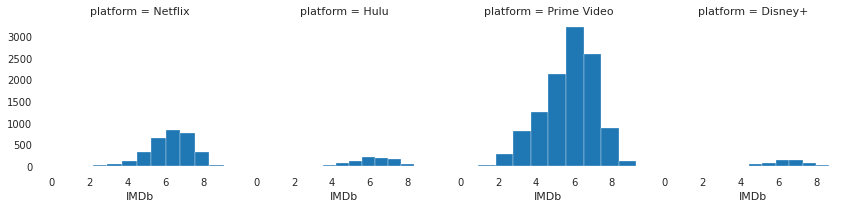The above plot shows the average IMDB rating distribution on each platform.## Runtime Per Platform Along with Age Group

In the "Working With Missing Values Section", I have dropped the Age column. For getting results of Runtime Per Platform Along with Age Group. I need to load the data again and apply the `melt()` function.

``````# Load dataset
df=df.iloc[:,1:]
df.ID = df.ID.astype("object")

# melting platform columns to create visualization
df2 = pd.melt(df, id_vars=["ID","Title","Year","Age","IMDb","Rotten Tomatoes","Type","Runtime"], var_name="platform")
df2 = df2[df2.value==1]
df2.drop(columns=["value"],axis=1,inplace=True)
``````

After loading the dataset again and performing melting, its time to generate a plot for runtime vs. streaming platform for different age groups.

``````# Total of runtime in different platform
ax = sns.barplot(x="platform", y="Runtime",hue="Age", estimator=sum, data=df2)
``````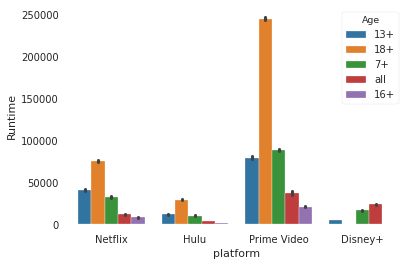The above plot shows that the total runtime on Prime Videos by 18+ age group users is way higher than compared to any other platform. You can interpret that the Prime Videos most of the content is focused on the 18+ Age group.

## Building a Recommender System

In the past few years, with the leap of YouTube, Walmart, Netflix, and many other such web-based services, recommender systems have created tremendous impact in the industry. From suggesting products/services to increasing companies value by online Ads-based monetization and matching the user's relevance and preference to make them buy. Recommender systems are irreplaceable in our daily web quests.

Generally, these are Math based frameworks focusing on suggesting products/services to end-users that are relevant to their needs and wants. For example, movies to watch, articles to read, products to buy, music to listen to, or anything depending on the domain. There are majorly three methods to build a Recommender Systems:

1. Content-Based Methods: Define a model for users or items it interacted with based on items feature set recommend other similar items to the users.
2. Collaborative Filtering Methods: Define a model for users and items interaction similarity score or User and User similarity score used for item recommendation.
3. Hybrid Methods: Use both content and collaborative methods to achieve a better result.

Let's first preprocess the dataset for the recommender system. First, you check the missing values:

``````# Reading Data Again
df=df.iloc[:,1:]
``````
``````#Finding Missing values in all columns
miss = pd.DataFrame(df.isnull().sum())
miss = miss.rename(columns={0:"miss_count"})
miss["miss_%"] = (miss.miss_count/len(df.ID))*100
miss
``````
``````#Dropping values with missing % more than 50%
df.drop(['Rotten Tomatoes', 'Age'], axis = 1, inplace=True)
# Dropping Na's from the following columns
df.dropna(subset=['IMDb','Directors', 'Genres', 'Country', 'Language', 'Runtime'],inplace=True)
df.reset_index(inplace=True,drop=True)
# converting into object type
df.ID = df.ID.astype("object")
df.Year = df.Year.astype("object")
``````

You will build two recommender system based on cosine similarity.

``````1. Using only the numerical variable
2. Using both numerical and categorical variable
``````

### Using only Numerical column

#### Step 1: Select the numerical variable

``````ndf = df.select_dtypes(include=['float64',"int64"])
``````

#### Step 2: Scaling the numerical variable using a min-max scaler to reduce model complexity and training time

``````#importing minmax scaler
from sklearn import preprocessing

# Create MinMaxScaler Object
scaler = preprocessing.MinMaxScaler(feature_range=(0, 1))

# Create dataframe after transformation
ndfmx = pd.DataFrame((scaler.fit_transform(ndf)))

# assign column names
ndfmx.columns=ndf.columns

# Show initial 5 records
``````

#### Step 3: Compute similarity score using cosine similarity

Now, you will compute the similarity score using cosine similarity.

``````# Import cosine similarity
from sklearn.metrics.pairwise import cosine_similarity

# Compute the cosine similarity
sig = cosine_similarity(ndfmx, ndfmx)

# Reverse mapping of indices and movie titles
indices = pd.Series(df.index, index=df['Title']).drop_duplicates()
``````
``````indices.head()
``````

#### Step 4: Writing a function to get recommendations based on the similarity score

• The function takes two arguments, movie title, and similarity scores. It searches the index of the title corresponding to the original_titles index in our series of indices.
• Get the pairwise similarity scores of all the movies.
• Sorting the similarity scores in descending order and converting them into a list.
• Getting the top 10 movies scores and indices and returning the title of the top 10 movies from our data frame.
``````def give_rec(title, sig=sig):

# Get the index corresponding to original_title
idx = indices[title]

# Get the pairwise similarity scores
sig_scores = list(enumerate(sig[idx]))

# Sort the movies
sig_scores = sorted(sig_scores, key=lambda x: x, reverse=True)

# Scores of the 10 most similar movies
sig_scores = sig_scores[1:11]

# Movie indices
movie_indices = [i for i in sig_scores]

# Top 10 most similar movies
return df['Title'].iloc[movie_indices]
``````
``````# Execute get_rec() function for getting recommendation
give_rec("The Matrix",sig = sig)
``````

Here, recommended movies are not up to the mark. The reason behind this poor result is that you are using only movie ratings, movie runtimes, and platform variables. You can improve this by using other information such as genre, directors, and country.

### Using Numerical and Textual columns

Since our last recommender system worked well but the recommendations were not up to the mark, so you will try a new, better approach to improve our results.

``````df.head()
``````

#### Step 1:

You will use textual columns into a single column then use tokenizer and TF-IDF Vectorizer to create a sparse matrix of all the words TF-IDF score. Then you will select and scale the numerical variables and add them into the sparse matrix. You need to perform the following steps for preprocessing:

• Selecting all object data types and storing them in a list.
• Removing ID and Title column.
• Joining all text/object columns using commas into a single column.
• Creating a tokenizer to remove unwanted elements from our data like symbols and numbers.
• Converting TfidfVector from the text After that we will do numerical columns pre-processing
• Selecting numerical variables into a data Frame
• Scaling Numerical variables using minmax scaler (0,1) range
• Adding numerical variables in the TF-IDF vectors sparse matrix using hstack function (hstack is used to add horizontal arrays into a sparse matrix).
``````#the function performs all the important preprocessing steps
def preprocess(df):
#combining all text columns
# Selecting all object data type and storing them in list
s = list(df.select_dtypes(include=['object']).columns)
# Removing ID and Title column
s.remove("Title")
s.remove("ID")
# Joining all text/object columns using commas into a single column
df['all_text']= df[s].apply(lambda x: ','.join(x.dropna().astype(str)),axis=1)

# Creating a tokenizer to remove unwanted elements from our data like symbols and numbers
token = RegexpTokenizer(r'[a-zA-Z]+')

# Converting TfidfVector from the text
cv = TfidfVectorizer(lowercase=True,stop_words='english',ngram_range = (1,1),tokenizer = token.tokenize)
text_counts= cv.fit_transform(df['all_text'])

# Aelecting numerical variables
ndf = df.select_dtypes(include=['float64',"int64"])

# Scaling Numerical variables
scaler = preprocessing.MinMaxScaler(feature_range=(0, 1))

# Applying scaler on our data and converting i into a data frame
ndfmx = pd.DataFrame((scaler.fit_transform(ndf)))
ndfmx.columns=ndf.columns

IMDb = ndfmx.IMDb.values[:, None]
X_train_dtm = hstack((text_counts, IMDb))
Netflix = ndfmx.Netflix.values[:, None]
X_train_dtm = hstack((X_train_dtm, Netflix))
Hulu = ndfmx.Hulu.values[:, None]
X_train_dtm = hstack((X_train_dtm, Hulu))
Prime = ndfmx["Prime Video"].values[:, None]
X_train_dtm = hstack((X_train_dtm, Prime))
Disney = ndfmx["Disney+"].values[:, None]
X_train_dtm = hstack((X_train_dtm, Disney))
Runtime = ndfmx.Runtime.values[:, None]
X_train_dtm = hstack((X_train_dtm, Runtime))
return X_train_dtm
``````

#### Step 2: Applying the function to our data and creating a sparse matrix

``````# Preprocessing data
mat =preprocess(df)
mat.shape
``````

#### Step 3: Again, applying Cosine Similarity to compute the similarity score

``````# using cosine similarity
from sklearn.metrics.pairwise import cosine_similarity

# Compute the sigmoid kernel
sig2 = cosine_similarity(mat, mat)

# Reverse mapping of indices and movie titles
indices = pd.Series(df.index, index=df['Title']).drop_duplicates()
``````

#### Step 4: Getting a recommendation from our improved system.

``````give_rec("The Matrix",sig=sig2)
``````

This time the recommender system works way better than the older system, which shows that by adding more relevant data like description text, a content-based recommender system can be improved significantly.

## Conclusion

Congratulations, you have made it to the end of this tutorial!

In this tutorial, you performed an exploratory analysis of the streaming platform movie dataset. You have explored missing values, individual distribution plots, and distribution of movies on each streaming platform. You have also discovered insights on genre, country, language, IMDB ratings, and movie runtime. Finally, you also have seen how to build a recommender system in python.

Check out DataCamp's Recommender Systems in Python tutorial.### .css-1531qan{-webkit-text-decoration:none;text-decoration:none;color:inherit;}Building Recommendation Engines in Python

Beginner
4 hr
7.1K
Learn to build recommendation engines in Python using machine learning techniques.
See Details

### Introduction to Python

Beginner
4 hr
4.9M
Master the basics of data analysis with Python in just four hours. This online course will introduce the Python interface and explore popular packages.

### Intermediate Python

Beginner
4 hr
947.9K
Level up your data science skills by creating visualizations using Matplotlib and manipulating DataFrames with pandas.
See More
Related

### How to Create a Data Analyst Resume

In this article, we'll discuss how to create a data analyst resume that will get you hired.

Matt Crabtree

7 min

### Building Your Data Science Portfolio with DataCamp Workspace (Part 1)

Learn how to build a comprehensive data science portfolio by exploring examples different examples, mastering tips to make your work stand out, and utilizing the DataCamp Workspace effectively to showcase your results.9 min

### Pandas 2.0: What’s New and Top Tips

Dive into pandas 2.0, the latest update of the essential data analysis library, with new features like PyArrow integration, nullable data types, and non-nanosecond datetime resolution for better performance and efficiency.### Building a Safer Internet with Data Science

Learn the key drivers of a data strategy that helps ensure online safety and consumer protection with Richard Davis, the Chief Data Officer at Ofcom, the UK’s government-approved regulatory and competition authority.

### Conda Cheat Sheet

In this cheat sheet, learn all about the basics of working with Conda. From managing and installing packages, to working with channels & environments, learn the fundamentals of the conda package management tool suite.

### Matplotlib time series line plot

This tutorial explores how to create and customize time series line plots in matplotlib.
See MoreSee More